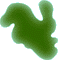List EffectsThis example shows how to list all the effects available and all the settings within those effects.
 List the EffectsWe iterate through all the Effects in the FX top level object. Every time we find an Effect we iterate through all the Settings within it. ```Set theFX = Server.CreateObject("ImageEffects.FX") For Each theEffect In theFX   Response.Write "
" & theEffect.Name & ": "   Response.Write theEffect.Description & "
"   For Each theSetting In theEffect     Response.Write theSetting.Name & " ["     Response.Write theSetting.Description & "] = "     Response.Write theSetting.Value & "
"   Next Next```
 ResultThis is an example of the kind of output you might expect. ```Median: Median Filter Width [Width of the filter in pixels] = 3 Height [Height of the filter in pixels] = 3 X [Horizontal center of the filter from top left] = 2 Y [Vertical center of the filter from top left] = 2``` `Sharpen: Basic Sharpen Convolution` ```Convolution: Generic Convolution Filter Width [Width of the filter in pixels] = 3 Height [Height of the filter in pixels] = 3 X [Horizontal center of the filter from top left] = 2 Y [Vertical center of the filter from top left] = 2 Number [The number of kernels] = 2 Values [List of convolution values] = -1,0,1,-2,0,2,-1,0,1,1,2,1,0,0,0,-1,-2,-1``` ```Gaussian Blur: Gaussian Blur Convolution Radius [Radius of the blur in pixels] = 1.2``` ```Laplacian: Laplacian of Gaussian Convolution Radius [Radius of the Gaussian in pixels] = 0.8``` ```Unsharp Mask: Unsharp Mask Sharpening Radius [Radius of the blur in pixels] = 1.5 Amount [The percentage of sharpness added] = 60 Threshold [Level difference at which sharpening will be done] = 0``` ```Despeckle: Despeckle Noise Reduction Threshold [Image complexity threshold for despeckling] = 20``` ```Brightness: Adjust Brightness Amount [Amount of brightness] = 0``` `Equalize: Equalize Brightness Levels` ```Contrast: Adjust Contrast Amount [Amount of contrast] = 0``` ```Levels: Adjust Black and White Levels Black Input [The input level that should be regarded as black] = 0 White Input [The input level that should be regarded as become white] = 255 Black Output [The level to define as black] = 0 White Output [The level to define as white] = 255``` ```AutoLevels: Automatic Color Level Adjustment Clip [The percentage of extreme values ignored] = 0.5``` ```Histogram: Histogram of Color Levels Red [The number of red values] = Green [The number of green values] = Blue [The number of blue values] = RGB [The combined RGB values] =``` ```Twirl: Twirl Distortion Angle [The amount of twirl in degrees] = 120 Extent [How far the effect extends] = 50 Speed [Speed versus quality tradeoff] = 8``` ```Pinch: Pinch distortion Amount [The amount of pinch] = 50 Extent [How far the effect extends] = 50 Speed [Speed versus quality tradeoff] = 3``` ```Ripple: Pond Ripple Distortion Height [The amplitude of the wave in pixels] = 10 Length [The wavelength in pixels] = 30 Phase [The phase of the wave in degrees] = 0 Speed [Speed versus quality tradeoff] = 6``` ```Wave: Random Wave Distortion Number [Number of waves] = 3 LengthMin [The lowest wavelength] = 40 LengthMax [The highest wavelength] = 60 HeightMin [The lowest amplitude] = 5 HeightMax [The highest amplitude] = 15 Speed [Speed versus quality tradeoff] = 6```